OS

Explainability

## Using Anchors to understand your model's results

Share

George Box, one of the greatest statistical minds of the 20th century, used to say “All models are wrong, but some are useful.” In machine learning, we may add that understanding how your model is wrong, its weaknesses and strengths, can make it even more useful. In this post, we introduce you to an algorithm that helps you conduct error analysis by giving you a better understanding of the local predictions of your models through simple decision rules (e.g. IF INCOME < 10K AND AGE > 25 THEN PREDICT 1).

Learn the secrets of performant and explainable ML

Be the first to know by subscribing to the blog. You will be notified whenever there is a new post.

## How Anchors works

The Anchors algorithm was proposed by Ribeiro, Singh, and Guestrin in 2018. These are the same authors who introduced the LIME algorithm in 2016 (check our previous blog post for a more detailed explanation) and the CheckList in 2020 (which we mentioned in this other blog post). Both Anchors and LIME use perturbation (shuffling of the data at a certain space) to generate local explanations for the given model. However, while LIME uses a linear model to approximate the results of a more complex model, Anchors defines an interval (coverage) for which a certain decision rule (the IF-ELSE statement we talked about) created by the model applies with a certain probability (precision).

But what exactly is an anchor? We can define it as a set of rules A(.) such that A(x) = 1 if x satisfies all of these rules. You can think of x here as a data point, or a row in our dataset, and A(x) as a simpler decision model that either classifies x to be from class 1 or 0. We want to have some notion of precision (how correct the explanation is, on average) and coverage (size of the region for which the explanation applies) to evaluate the different A(.) one can come up with. Here, the authors define a minimum precision threshold τ such that all samples from the conditional distribution around the perturbation when A holds have a probability τ of being predicted to be in class 1.

While one can find multiple anchors A that satisfy this threshold τ, the optimal anchor will be the one that maximizes our coverage, that is, the one that can be used to explain a larger set of the data. Thus, the problem of finding the optimal A(.) can be rewritten as the maximization problem:

$\max \text{cov}(A), A~ s.t. ~ \text{prec}(A) \geq \tau$

where prec(A) is the precision of A and cov(A) is the coverage of A.

Note the existence of a trade-off between precision and coverage. All else equal, an anchor defined by more rules will have a higher precision but will also have a smaller coverage since it will be too specific and apply to very few observations. As an illustration, check the image below. D is the perturbation space (circle), the anchor covers the area defined by the square, and the different shades represent observations in different classes. Our problem is to find the anchor with the highest area such that one shade covers at least τ% of its area.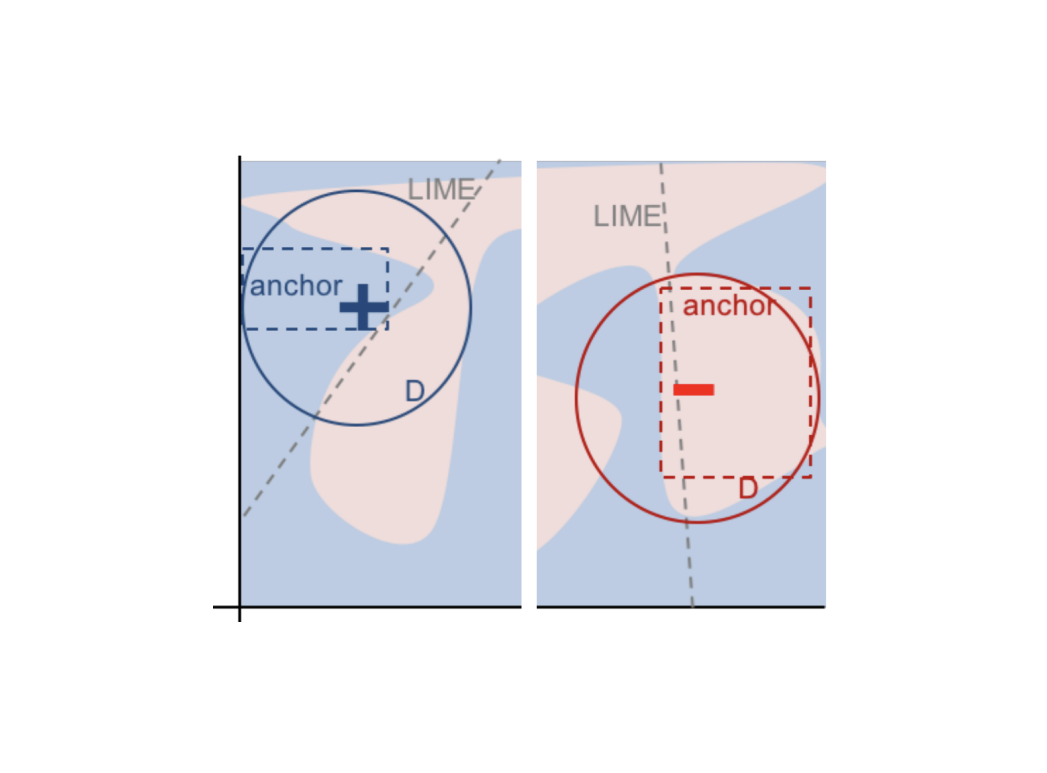Source: Anchors paper.

## Breast cancer detection with Anchors

Let’s look into an application of Anchors to help explain the predictions made by an XGBoost on the Breast Cancer Diagnostic Wisconsin dataset. The objective here is to classify malign and benign breast tumors using physical measurements such as area, radius, perimeter, and others. This is a great application of Anchors not only because of the dimensionality and non-linearity of the problem but also because explaining a model’s output here can be useful in convincing doctors and patients of a misdiagnosis and preventing a medical error.

After training the model and running the Anchors algorithm with a minimum precision threshold of 0.9, we can start our exploration. To investigate the decisions made by the black-box model, let’s first check the number of times our trees used each feature as a simple method to grasp its feature importances.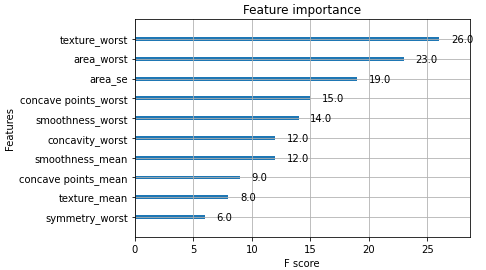We now have some understanding of which features are being used to classify the tumors and we can use Anchors to visualize the ”reasoning” of our model for observations that were near the decision boundary.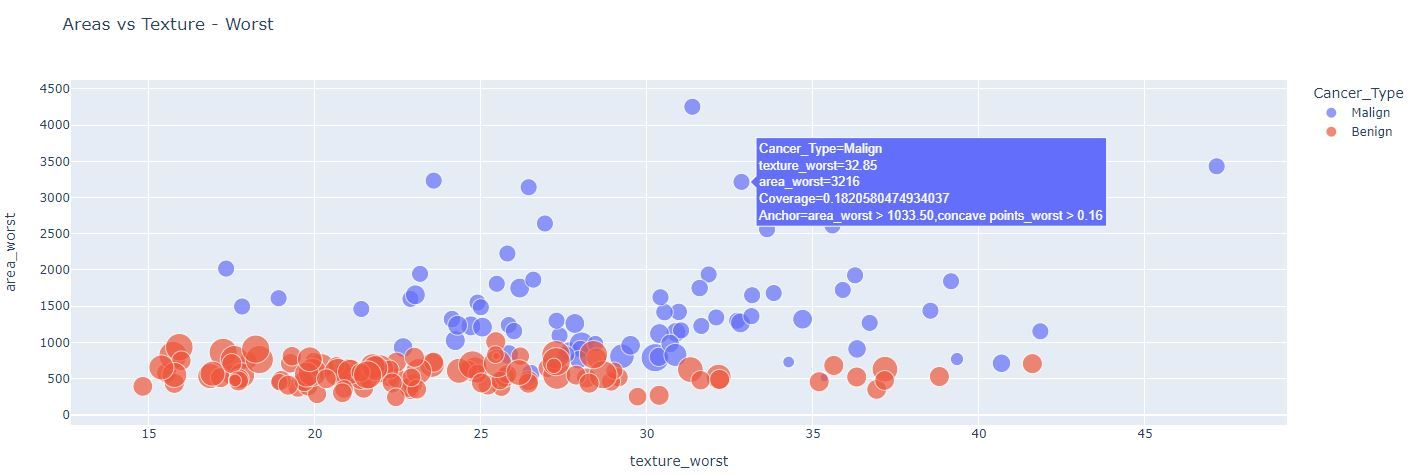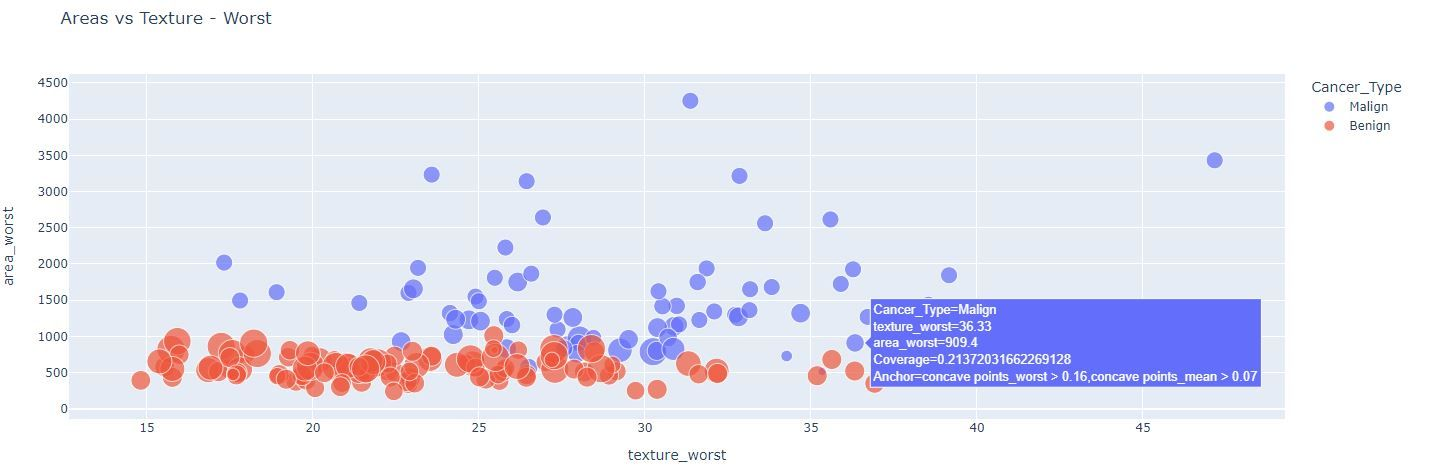The two figures above depict the anchor explanations for points far away and close to the decision boundary, respectively.

From the plot, it seems that area_worst is enough to separate most of the observations in the two classes. In fact, when we hover our mouse over the observations, we see the anchor explanations (i.e., the inequalities separated by the comma) frequently contain the variable area_worst. However, there are a few observations with area_worst in between 700 and 1100 for which area_worst by itself cannot explain the divergence in classification. For these data points, our anchors show the rules tend to use variables related to the concavity of the tumors. We can check this by subsetting our data to restrict it to the specific range of area worst where the decision boundary lies and observing how concave points worst and concave points mean relate to each other.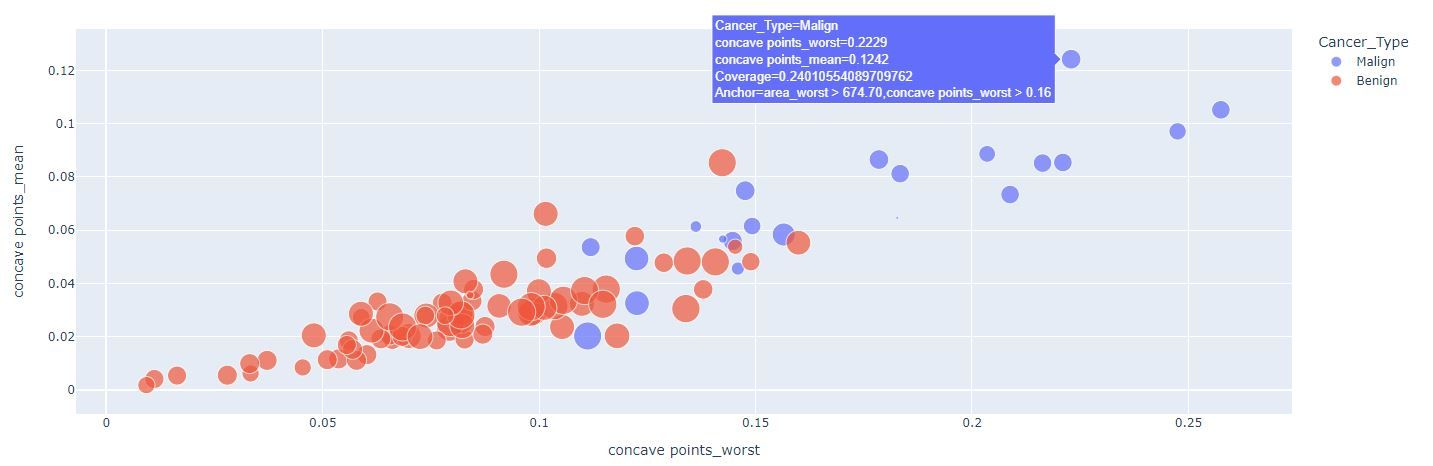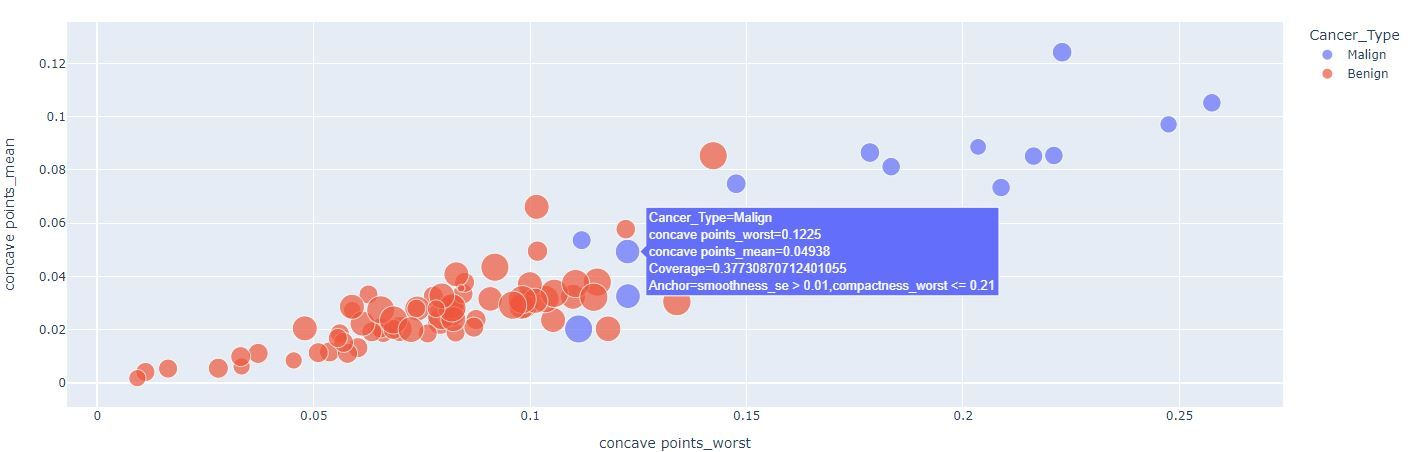Again, the figures above show the anchor explanations for points far away and close to the decision boundary, respectively.

Now it’s easy to see that our model uses higher concavity to identify smaller malign tumors. Nevertheless, there are still some mid-concavity tumors that are classified as malign. You can use your mouse to hover through the data points and see anchor results for these data points - smoothness and compactness seem to play a role in defining the class of these observations. We can keep applying this reasoning, but note that the coverage of these explanations (size of the points here) tends to get smaller.

This process of interpreting the results of the anchor algorithm allows an analyst to understand the model’s predictions locally while being aware of its coverage/limitations. One can also change the chosen precision threshold, since this was a somewhat arbitrary choice, and have less specific explanations. We encourage you to apply Anchors to explain the predictions of your models. Let us know what you find out!